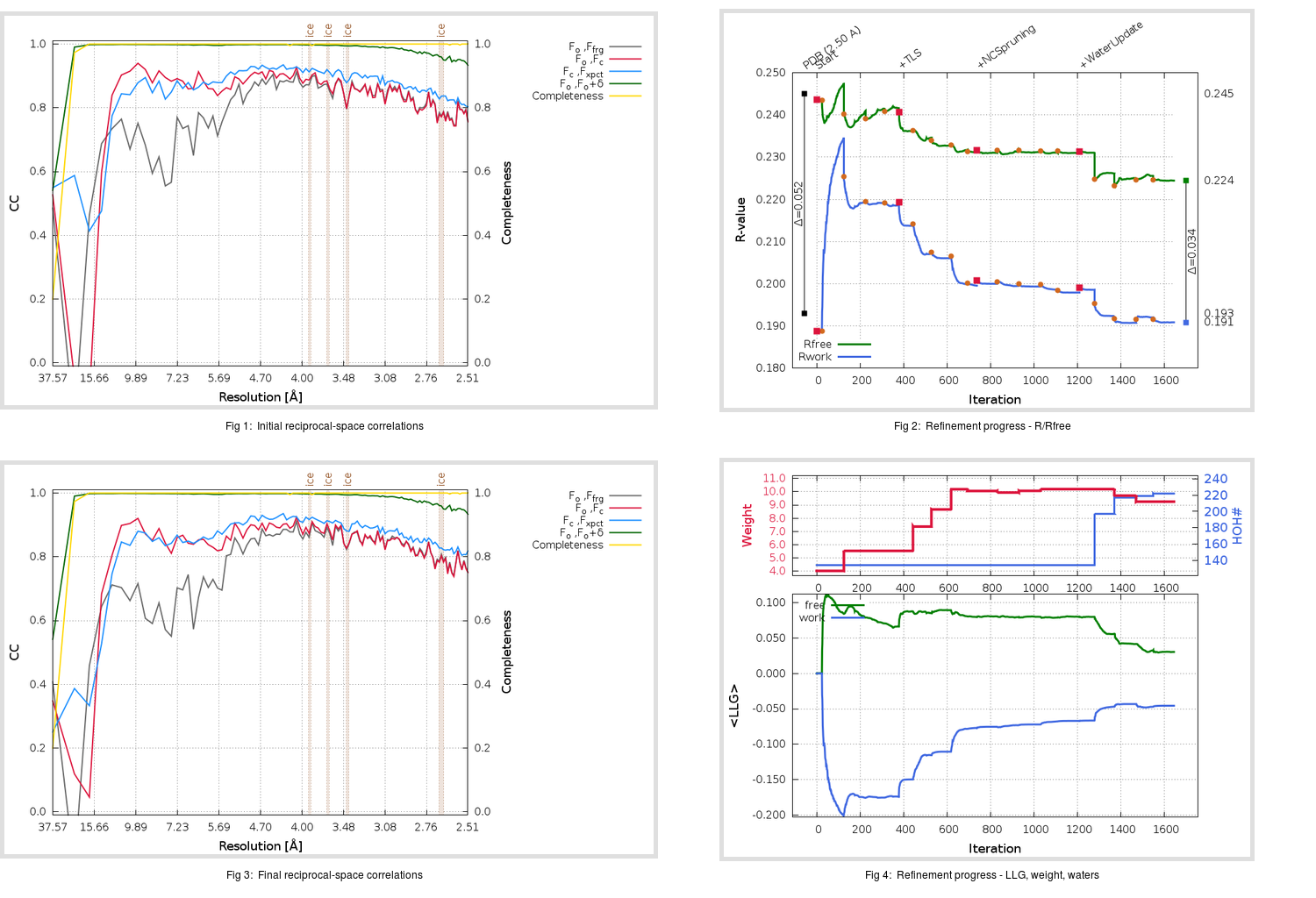Content:

## Deposited

` `
 Date deposited Date data collection Resolution R, Rfree 20111231 20091014 2.50 0.1880 0.2450

Molprobity (CCP4 7.0 version) summary:

```Ramachandran outliers =   0.99 %
favored =  93.22 %
Rotamer outliers      =   2.85 %
C-beta deviations     =     0
Clashscore            =  10.17
RMS(bonds)            =   0.0078
RMS(angles)           =   1.15
MolProbity score      =   2.31
Resolution            =   2.50
R-work                =   0.1880
R-free                =   0.2450
```

```Number of waters      =   134

<B> (all atoms) =   36.44 ( sd =   12.00 ) for       4899 non-hydrogen atoms
<B>   (protein) =   36.46 ( sd =   12.11 ) for       4743 non-hydrogen atoms
<B>     (water) =   34.96 ( sd =    7.50 ) for        134 non-hydrogen atoms
<B>    (others) =   42.74 ( sd =    6.67 ) for         22 non-hydrogen atoms

B min/max       (all non-hydrogen atoms) =   15.48 /   89.08
B min/max   (protein non-hydrogen atoms) =   15.48 /   89.08
B min/max     (water non-hydrogen atoms) =   17.70 /   55.17
B min/max     (other non-hydrogen atoms) =   30.61 /   56.06
```

## BUSTER (re-)refinement

` `

Molprobity (CCP4 7.0 version) summary:

```Ramachandran outliers =   0.00 %
favored =  96.69 %
Rotamer outliers      =   3.61 %
C-beta deviations     =     0
Clashscore            =   4.24
RMS(bonds)            =   0.0115
RMS(angles)           =   1.58
MolProbity score      =   1.84
Resolution            =   2.50
R-work                =   0.1909
R-free                =   0.2246
```

```Number of waters      =   222

<B> (all atoms) =   40.59 ( sd =   13.60 ) for       4987 non-hydrogen atoms
<B>   (protein) =   40.49 ( sd =   13.70 ) for       4743 non-hydrogen atoms
<B>     (water) =   41.05 ( sd =   10.53 ) for        222 non-hydrogen atoms
<B>    (others) =   57.29 ( sd =    8.24 ) for         22 non-hydrogen atoms

B min/max       (all non-hydrogen atoms) =   17.30 /  109.12
B min/max   (protein non-hydrogen atoms) =   17.30 /  109.12
B min/max     (water non-hydrogen atoms) =   18.20 /   71.52
B min/max     (other non-hydrogen atoms) =   46.91 /   67.85
```

Refinement progression:Results:

` `
 File Remark 3VB6_aB_refine.01_04_refine.pdb.gz exact refinement commands are in header 3VB6_aB_refine.01_04_refine.mtz.gz including original deposited data and several re-refinement map coefficients 3VB6_aB_refine.01_04_BUSTER_model.cif.gz including any non-standard compound restraints 3VB6_aB_refine.01_04_BUSTER_refln.cif.gz